Home
Hostname: page-component-568f69f84b-xr9nb Total loading time: 0.281 Render date: 2021-09-17T07:58:14.260Z Has data issue: true Feature Flags: { "shouldUseShareProductTool": true, "shouldUseHypothesis": true, "isUnsiloEnabled": true, "metricsAbstractViews": false, "figures": true, "newCiteModal": false, "newCitedByModal": true, "newEcommerce": true, "newUsageEvents": true }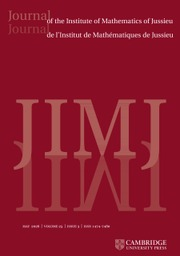Journal of the Institute of Mathematics of Jussieu

# Uniform Sobolev estimates for non-trapping metrics

Published online by Cambridge University Press:  07 October 2013

## Abstract

We prove uniform Sobolev estimates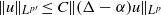$\Vert u\Vert _{{L}^{p\prime } } \leq C\Vert (\Delta - \alpha )u\Vert _{{L}^{p} }$ for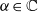$\alpha \in \mathbb{C}$ and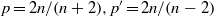$p= 2n/ (n+ 2), {p}^{\prime } = 2n/ (n- 2)$ on non-trapping asymptotically conic manifolds of dimension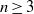$n\geq 3$ , generalizing to non-constant coefficient Laplacians a result of Kenig, Ruiz and Sogge .

Type
Research Article
Information

## Access options

Get access to the full version of this content by using one of the access options below. (Log in options will check for institutional or personal access. Content may require purchase if you do not have access.)

## References

Bourgain, J., Shao, P., Sogge, C. D. and Yao, X., On${L}^{p}$ -resolvent estimates and the density of eigenvalues for compact Riemannian manifolds (arXiv:1204.3927).Google Scholar
Cheng, S. Y, Li, P. and Yau, S.-T., On the upper estimate of the heat kernel of a complete Riemannian manifold, Amer. J. Math. 103 (1981), 10211063.CrossRefGoogle Scholar
Datchev, K., Local smoothing for scattering manifolds with hyperbolic trapped sets, Commun. Math. Phys. 286 (3) (2009), 837850.CrossRefGoogle Scholar
Dimassi, M. and Sjöstrand, J., Spectral asymptotics in the semi-classical limit, London Mathematical Society Lecture Note Series, Volume 268, 1999), xii+227 pp.CrossRefGoogle Scholar
Dos Santos Ferreira, D., Kenig, C. E. and Salo, M., On${L}^{p}$ resolvent estimates for Laplace–Beltrami operators on compact manifolds, Forum Math. to appear.Google Scholar
García-Cuerva, J. and Gatto, A. E., Boundedness properties of fractional integral operators associated to non-doubling measures, Studia Math. 162 (2004), 245261.CrossRefGoogle Scholar
Guillarmou, C. and Hassell, A., Resolvent at low energy and Riesz transform for Schrödinger operators on asymptotically conic manifolds, I. Math. Ann. 341 (4) (2008), 859896.CrossRefGoogle Scholar
Guillarmou, C., Hassell, A. and Sikora, A., Resolvent at low energy III: the spectral measure, Trans. Amer. Math. Soc. 365 (2013), 61036148.CrossRefGoogle Scholar
Guillarmou, C., Hassell, A. and Sikora, A., Restriction and spectral multiplier theorems on asymptotically conic manifolds, Anal. PDE 6 (4) (2013), 893950.CrossRefGoogle Scholar
Hassell, A. and Vasy, A., The resolvent for Laplace-type operators on asymptotically conic spaces, Ann. Inst. Fourier (Grenoble) 51 (5) (2001), 12991346.CrossRefGoogle Scholar
Hassell, A. and Wunsch, J., The semiclassical resolvent and the propagator for non-trapping scattering metrics, Adv. Math. 217 (2) (2008), 586682.CrossRefGoogle Scholar
Ionescu, A. D. and Jerison, D., On the absence of positive eigenvalues of Schrödinger operators with rough potentials, Geom. Funct. Anal. 13 (5) (2003), 10291081.CrossRefGoogle Scholar
Kenig, C. E., Ruiz, A. and Sogge, C. D., Uniform Sobolev inequalities and unique continuation for second order constant coefficient differential operators, Duke Math. J. 55 (1987), 329347.CrossRefGoogle Scholar
Koch, H. and Tataru, D., Carleman estimates and absence of embedded eigenvalues, Commun. Math. Phys. 267 (2) (2006), 419449.CrossRefGoogle Scholar
Melrose, R. B., The Atiyah–Patodi–Singer index theorem. (AK Peters, Wellesley, 1993).Google Scholar
Melrose, R. B., Spectral and scattering theory for the Laplacian on asymptotically Euclidean spaces, in Spectral and scattering theory (Sanda, 1992), Lecture Notes in Pure and Appl. Math., Volume 161, pp. 85130 (Dekker, New York, 1994).Google Scholar
Melrose, R. B. and Zworski, M., Scattering metrics and geodesic flow at infinity, Invent. Math. 124 (1–3) (1996), 389436.CrossRefGoogle Scholar
Rodnianski, I. and Tao, T., Effective limiting absorption principles, and applications (arXiv:1105.0873).Google Scholar
Seeley, R. T., Complex powers of an elliptic operator, in Singular integrals (Proc. Sympos. Pure Math., Chicago, Ill., 1966), pp. 288307 (Amer. Math. Soc., Providence, RI, 1967).Google Scholar
Shen, Z., On absolute continuity of the periodic Schrödinger operators, Internat. Math. Res. Not. 1 (2001), 131.CrossRefGoogle Scholar
Stein, E., Harmonic analysis, in Real variable methods, orthogonality and oscillatory integrals. (Princeton University Press, 1993).CrossRefGoogle Scholar
Varopoulos, N., Hardy–Littlewood Sobolev for semigroups, J. Funct. Anal. 63 (2) (1985), 240260.CrossRefGoogle Scholar
Vasy, A. and Zworski, M., Semi-classical estimates in asymptotically Euclidean scattering, Commun. Math. Phys. 212 (2000), 205217.CrossRefGoogle Scholar
Wunsch, J. and Zworski, M., Distribution of resonances for asymptotically Euclidean manifolds, J. Differential Geom. 55 (1) (2000), 4382.CrossRefGoogle Scholar
Zworski, M., Semiclassical analysis, Graduate Studies in Mathematics, Volume 138 (AMS, 2012).CrossRefGoogle Scholar
11
Cited by

# Send article to Kindle

Note you can select to send to either the @free.kindle.com or @kindle.com variations. ‘@free.kindle.com’ emails are free but can only be sent to your device when it is connected to wi-fi. ‘@kindle.com’ emails can be delivered even when you are not connected to wi-fi, but note that service fees apply.

Find out more about the Kindle Personal Document Service.

Uniform Sobolev estimates for non-trapping metrics
Available formats
×

# Send article to Dropbox

To send this article to your Dropbox account, please select one or more formats and confirm that you agree to abide by our usage policies. If this is the first time you use this feature, you will be asked to authorise Cambridge Core to connect with your <service> account. Find out more about sending content to Dropbox.

Uniform Sobolev estimates for non-trapping metrics
Available formats
×

# Send article to Google Drive

To send this article to your Google Drive account, please select one or more formats and confirm that you agree to abide by our usage policies. If this is the first time you use this feature, you will be asked to authorise Cambridge Core to connect with your <service> account. Find out more about sending content to Google Drive.

Uniform Sobolev estimates for non-trapping metrics
Available formats
×
×